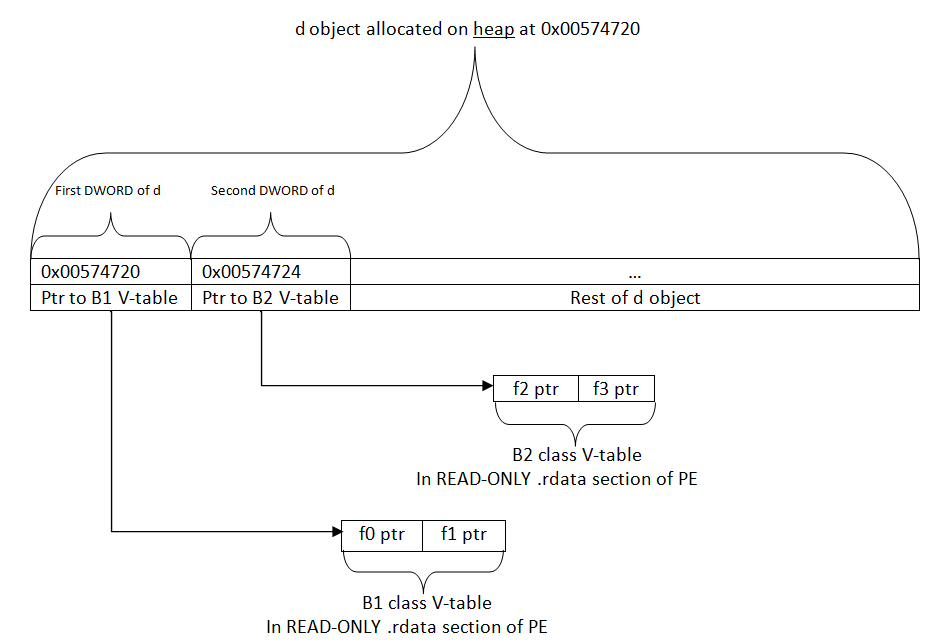# Attacking V-Table Pointers

A common attack vector for software written in C++ is V-table pointer overwrites. When C++ objects are allocated on the heap, such as when the “new” keyword is used, they often get put next to other objects that are also on the heap. If there is an unbounded write to one of the objects on the heap before an object using V-tables, this type of attack is feasible.

Windows has mitigations in its userland heap manager that can make it difficult to guess which objects will be next to each other on the heap. This means that even if an attacker knows that there is an unbounded write to an object on the heap, the attacker would not know what object is right after it on the heap, making it much more difficult to exploit reliably.

The following example code uses Virtual functions, which imply V-table usage when compiled with the Microsoft Visual C++ compiler:

``` /*
the following class definitions were modified from
http://en.wikipedia.org/wiki/Virtual_function_table
*/

#include <iostream>
using namespace std;

class B1    //base class
{
public:
virtual void f0() {}
virtual void f1() {}
};

class B2    //base class
{
public:
virtual void f2() {}
virtual void f3() {}
};

class D : public B1, public B2 {    //derived class inherits both base classes
public:
void d() {}
void f0() {}  // override B1::f0()
void f1() {}  // override B1::f1()
void f2() {}  // override B2::f2()
void f3() {}  // override B2::f3()
};

int main(int argc, char* argv[])
{
B2 *b2 = new B2();
D  *d  = new D();
d->f0();    //vtable lookup
d->f1();    //vtable lookup
d->f2();    //vtable lookup
d->f3();    //vtable lookup
}

Below is the relevant assembly code of the above C++ code showing how virtual functions are accessed in objects that make use of virtual functions:

d->f0();    //V-table lookup
mov         eax,dword ptr [ebp-14h]//eax=address of d object
mov         edx,dword ptr [eax]    //edx=first dword in d object(pointer to B1 V-table)
mov         eax,dword ptr [edx]    //eax=first entry in B1 V-Table(address of f0)
call        eax

d->f1();    //V-table lookup
mov         eax,dword ptr [ebp-14h]//eax=address of d object
mov         edx,dword ptr [eax]    //edx=first dword in d object(pointer to B1 V-table)
mov         eax,dword ptr [edx+4]  //eax=second entry in B1 V-Table(address of f1)
call        eax

d->f2();    //V-table lookup
mov         eax,dword ptr [ebp-14h]//eax=address of d object
mov         edx,dword ptr [eax+4]  //edx=second dword in d object(pointer to B2 V-table)
mov         eax,dword ptr [edx]    //eax=first entry in B2 V-table(address of f2)
call        eax

d->f3();    //V-table lookup
mov         eax,dword ptr [ebp-14h]//eax=address of d object
mov         edx,dword ptr [eax+4]  //edx=second dword in d object(pointer to B2 V-table)
mov         eax,dword ptr [edx+4]  //eax=second entry in B2 V-table(address of f3)
call        eax```

The common pattern in all of these virtual function lookups is as follows:

1. Dereference the object pointer which contains the V-table.
2. Dereference the relevant V-Table pointer within the object from step 1.
3. Dereference the relevant function pointer inside the V-table from step 2.
4. Call the function found in step 3.

In Windbg, we can verify that d was indeed allocated on the heap because our local variables are:

In Windbg, we can verify that d was indeed allocated on the heap because our local variables are:

```0:000> dv
argc = 0n1
argv = 0x00574660
d = 0x00574720
b2 = 0x005746e0```

```0:000> !heap -p -a 0x00574720

_HEAP @ 570000

HEAP_ENTRY Size Prev Flags    UserPtr UserSize - state
005746f8 0009 0000     00574700    0002c - (busy)```

Below is a graphical depiction of the relationship between our heap objects and the V-tables they reference.Normal V-table layout

If we wanted to exploit a V-table pointer overwrite and highjack a call to d->f1(), we have to make sure our “fake V-table” and attacker code is in place before executing the call. For this example let’s assume the “fake V-table” is at 0xDEADBEEF and our attacker code is at 0x41414141. This can be achieved by memory spraying, which would ensure that the following predicates would be true:

2. The DWORD at 4 bytes past 0xDEADBEEF(doing the math, that would just be 0xDEADBEF3) is the address of attacker code that we want to execute.
3. Our attacker code exists at 0x41414141.

We would need to overwrite the pointer(stored in the heap-allocated object) to the B1 class V-table with the value 0xDEADBEEF. In the following example, we overwrote the pointer(stored at 0x00574720) to the B1 V-table with the value 0xDEADBEEF.

Now, if a call to d->f1() happened, the follow sequence of events would occur:

```    d->f1();    //V-table lookup
mov         eax,dword ptr [ebp-14h]//eax=address of d object
mov         edx,dword ptr [eax]    //edx=first dword in d object(our 0xDEADBEEF value)
mov         eax,dword ptr [edx+4]  //eax=0x41414141
call        eax                    //call into our attacker code instead of d->f1()```Overwritten V-table pointers with sprayed fake V-table and attacker code

By: Neil Sikka

Save

Posted On : 2013/02/11

## Blog Archive

WordPress Lightbox